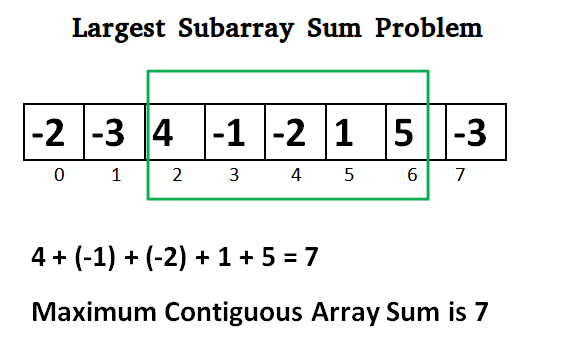# Java Program for Largest Sum Contiguous Subarray

• Last Updated : 29 Nov, 2021

Write an efficient program to find the sum of contiguous subarray within a one-dimensional array of numbers that has the largest sum.```Initialize:
max_so_far = INT_MIN
max_ending_here = 0

Loop for each element of the array
(a) max_ending_here = max_ending_here + a[i]
(b) if(max_so_far < max_ending_here)
max_so_far = max_ending_here
(c) if(max_ending_here < 0)
max_ending_here = 0
return max_so_far```

Explanation:
The simple idea of Kadane's algorithm is to look for all positive contiguous segments of the array (max_ending_here is used for this). And keep track of maximum sum contiguous segment among all positive segments (max_so_far is used for this). Each time we get a positive-sum compare it with max_so_far and update max_so_far if it is greater than max_so_far

```    Lets take the example:
{-2, -3, 4, -1, -2, 1, 5, -3}

max_so_far = max_ending_here = 0

for i=0,  a =  -2
max_ending_here = max_ending_here + (-2)
Set max_ending_here = 0 because max_ending_here < 0

for i=1,  a =  -3
max_ending_here = max_ending_here + (-3)
Set max_ending_here = 0 because max_ending_here < 0

for i=2,  a =  4
max_ending_here = max_ending_here + (4)
max_ending_here = 4
max_so_far is updated to 4 because max_ending_here greater
than max_so_far which was 0 till now

for i=3,  a =  -1
max_ending_here = max_ending_here + (-1)
max_ending_here = 3

for i=4,  a =  -2
max_ending_here = max_ending_here + (-2)
max_ending_here = 1

for i=5,  a =  1
max_ending_here = max_ending_here + (1)
max_ending_here = 2

for i=6,  a =  5
max_ending_here = max_ending_here + (5)
max_ending_here = 7
max_so_far is updated to 7 because max_ending_here is
greater than max_so_far

for i=7,  a =  -3
max_ending_here = max_ending_here + (-3)
max_ending_here = 4```

Program:

## Java

 `import` `java.io.*;``// Java program to print largest contiguous array sum``import` `java.util.*;`` ` `class` `Kadane``{``    ``public` `static` `void` `main (String[] args)``    ``{``        ``int` `[] a = {-``2``, -``3``, ``4``, -``1``, -``2``, ``1``, ``5``, -``3``};``        ``System.out.println(``"Maximum contiguous sum is "` `+``                                       ``maxSubArraySum(a));``    ``}`` ` `    ``static` `int` `maxSubArraySum(``int` `a[])``    ``{``        ``int` `size = a.length;``        ``int` `max_so_far = Integer.MIN_VALUE, max_ending_here = ``0``;`` ` `        ``for` `(``int` `i = ``0``; i < size; i++)``        ``{``            ``max_ending_here = max_ending_here + a[i];``            ``if` `(max_so_far < max_ending_here)``                ``max_so_far = max_ending_here;``            ``if` `(max_ending_here < ``0``)``                ``max_ending_here = ``0``;``        ``}``        ``return` `max_so_far;``    ``}``}`

Output:

`Maximum contiguous sum is 7`

Another approach:

## Java

 `static` `int` `maxSubArraySum(``int` `a[],``int` `size) ``{ ``     ` `    ``int` `max_so_far = a[``0``], max_ending_here = ``0``; `` ` `    ``for` `(``int` `i = ``0``; i < size; i++) ``    ``{ ``        ``max_ending_here = max_ending_here + a[i];``        ``if` `(max_ending_here < ``0``) ``            ``max_ending_here = ``0``; ``         ` `        ``/* Do not compare for all``           ``elements. Compare only ``           ``when max_ending_here > 0 */``        ``else` `if` `(max_so_far < max_ending_here) ``            ``max_so_far = max_ending_here; ``         ` `    ``} ``    ``return` `max_so_far; ``} `` ` `// This code is contributed by ANKITRAI1`

Time Complexity: O(n)

Following is another simple implementation suggested by Mohit Kumar. The implementation handles the case when all numbers in the array are negative.

## Java

 `// Java program to print largest contiguous``// array sum``import` `java.io.*;`` ` `class` `GFG {`` ` `    ``static` `int` `maxSubArraySum(``int` `a[], ``int` `size)``    ``{``    ``int` `max_so_far = a[``0``];``    ``int` `curr_max = a[``0``];`` ` `    ``for` `(``int` `i = ``1``; i < size; i++)``    ``{``           ``curr_max = Math.max(a[i], curr_max+a[i]);``        ``max_so_far = Math.max(max_so_far, curr_max);``    ``}``    ``return` `max_so_far;``    ``}`` ` `    ``/* Driver program to test maxSubArraySum */``    ``public` `static` `void` `main(String[] args)``    ``{``    ``int` `a[] = {-``2``, -``3``, ``4``, -``1``, -``2``, ``1``, ``5``, -``3``};``    ``int` `n = a.length;   ``    ``int` `max_sum = maxSubArraySum(a, n);``    ``System.out.println(``"Maximum contiguous sum is "` `                       ``+ max_sum);``    ``}``}`` ` `// This code is contributed by Prerna Saini`

Output:

`Maximum contiguous sum is 7`

To print the subarray with the maximum sum, we maintain indices whenever we get the maximum sum.

## Java

 `// Java program to print largest ``// contiguous array sum``class` `GFG {`` ` `    ``static` `void` `maxSubArraySum(``int` `a[], ``int` `size)``    ``{``        ``int` `max_so_far = Integer.MIN_VALUE,``        ``max_ending_here = ``0``,start = ``0``,``        ``end = ``0``, s = ``0``;`` ` `        ``for` `(``int` `i = ``0``; i < size; i++) ``        ``{``            ``max_ending_here += a[i];`` ` `            ``if` `(max_so_far < max_ending_here) ``            ``{``                ``max_so_far = max_ending_here;``                ``start = s;``                ``end = i;``            ``}`` ` `            ``if` `(max_ending_here < ``0``) ``            ``{``                ``max_ending_here = ``0``;``                ``s = i + ``1``;``            ``}``        ``}``        ``System.out.println(``"Maximum contiguous sum is "` `                           ``+ max_so_far);``        ``System.out.println(``"Starting index "` `+ start);``        ``System.out.println(``"Ending index "` `+ end);``    ``}`` ` `    ``// Driver code``    ``public` `static` `void` `main(String[] args)``    ``{``        ``int` `a[] = { -``2``, -``3``, ``4``, -``1``, -``2``, ``1``, ``5``, -``3` `};``        ``int` `n = a.length;``        ``maxSubArraySum(a, n);``    ``}``}`` ` `// This code is contributed by  prerna saini`

Output:

```Maximum contiguous sum is 7
Starting index 2
Ending index 6```

Kadane's Algorithm can be viewed both as a greedy and DP. As we can see that we are keeping a running sum of integers and when it becomes less than 0, we reset it to 0 (Greedy Part). This is because continuing with a negative sum is way more worse than restarting with a new range. Now it can also be viewed as a DP, at each stage we have 2 choices: Either take the current element and continue with previous sum OR restart a new range. These both choices are being taken care of in the implementation.

Time Complexity: O(n)

Auxiliary Space: O(1)

Now try the below question
Given an array of integers (possibly some elements negative), write a C program to find out the *maximum product* possible by multiplying 'n' consecutive integers in the array where n ≤ ARRAY_SIZE. Also, print the starting point of the maximum product subarray.